ID 772987
Date 12/04/2020
Public

## UniformBits (VSL_RNG_METHOD_UNIFORMBITS_STD)

A random number generator of uniform distribution that produces an integer (non-normalized to the interval (0, 1)) sequence. You can identify the underlying BRNG by passing the random stream descriptor stream as a parameter. Then UniformBits function calls integer implementation of this basic generator.

Basic generators differ in bit capacity and structure of the integer output, therefore you should interpret the output integer array of the function viRngUniformBits correctly. The following list provides rules for interpreting 32-bit integer output r[i] for each VS BRNG.

### MCG31m1

Integer Recurrence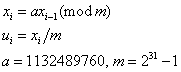Interpretation of 32-bit integer output array r[i] after calling viRngUniformBits### R250

Integer Recurrence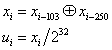Interpretation of 32-bit integer output array r[i] after calling viRngUniformBits### MRG32k3a

Integer RecurrenceInterpretation of 32-bit integer output array r[i] after calling viRngUniformBits### MCG59

Integer RecurrenceInterpretation of 32-bit integer output array r[i] after calling viRngUniformBits### WH

Integer Recurrence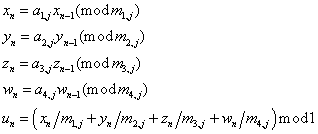Interpretation of 32-bit integer output array r[i] after calling viRngUniformBits### MT19937

Integer Recurrence,,,,

where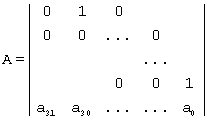,

with.

Interpretation of 32-bit integer output array r[i] after calling viRngUniformBits### MT2203

Integer Recurrence,

where,

with,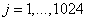.

Interpretation of 32-bit integer output array r[i] after calling viRngUniformBits### SFMT19937

Integer RecurrenceInterpretation of 32-bit integer output array r[i] after calling viRngUniformBits

r[i] = wi/4(i % 4)

### SOBOL

Integer Recurrence,

where,and s is the dimension of quasi-random vector.

Interpretation of 32-bit integer output array r[i] after calling viRngUniformBits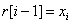### NIEDERR

Integer Recurrence,

where,and s is the dimension of quasi-random vector.

Interpretation of 32-bit integer output array r[i] after calling viRngUniformBits### PHILOX4X32X10

Integer Recurrence

cn = cn-1 + 1

wn = f(cn)

f(c) is computed as follows:k00 = k0

k10 = k1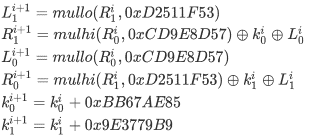f(c)=, N= 10

Interpretation of 32-bit integer output array r[i] after calling viRngUniformBits

r[i] = wi/4(i % 4)

wi(k) is the k-th 32-bit integer in quadruple wn, k= 0, 1, 2, 3

### ARS5

Integer Recurrence

cn = cn-1 + 1

wn = f(cn)

f(c) is computed as follows:

c0 = c xor kand k0 = k

ci+1= SubBytes(c)

ci+1= ShiftRows(ci+1)

ci+1= MixColumns(ci+1), omitted if i + 1 = N

Lo(ki+1)=Lo(k)+ 0x9E3779B97F4A7C15

Hi(ki+1)= Hi(k)+ 0xBB67AE8584CAA73B

f(c) = cN, N= 5

Interpretation of 32-bit integer output array r[i] after calling viRngUniformBits

r[i] = wi/4(i % 4)

wi(k) is the k-th 32-bit integer in quadruple wn, k= 0, 1, 2, 3

### NON-DETERMINISTIC

Integer Recurrence

Non-deterministic random generator [BMT] available in the latest Intel® CPUs [AVX].

Interpretation of 32-bit integer output array r[i] after calling viRngUniformBitsNOTE:
1. Lo(x) means obtaining lower 32 bits of the 64-bit unsigned integer x, that is, Lo(x) = xmod232.

2. Hi(x) means obtaining upper 32 bits of the 64-bit unsigned integer x, that is, Hi(x) =x/232⌋.

3. ABCD means a 128-bit number composed of four 32-bit numbers A, B, C and D, that isSo, when you generate an integer sequence of n elements, the output array r[i] of the function viRngUniformBits comprises:

1. n elements for the basic generators MCG31m1, R250, MRG32k3a, MT19937, MT2203, SOBOL, NIEDERR, Philox4x32-10 and ARS5.

2. 2n elements for the basic generator MCG59.

3. 4n elements for the basic generators WH and SFMT19937.

You may use the integer output, in particular, for fast generation of bit vectors. However, in this case some bits (or groups of them) might be non-random. For example, lower bits produced by linear congruential generators are less random than their higher bits. Note that quasi-random numbers are not random at all. Thoroughly check the integer output bits and bit groups for randomness before forming bit vectors from r[i] array.

See Intel® oneAPI Math Kernel Library Vector Statistics Random Number Generator Performance Data for test results summary.